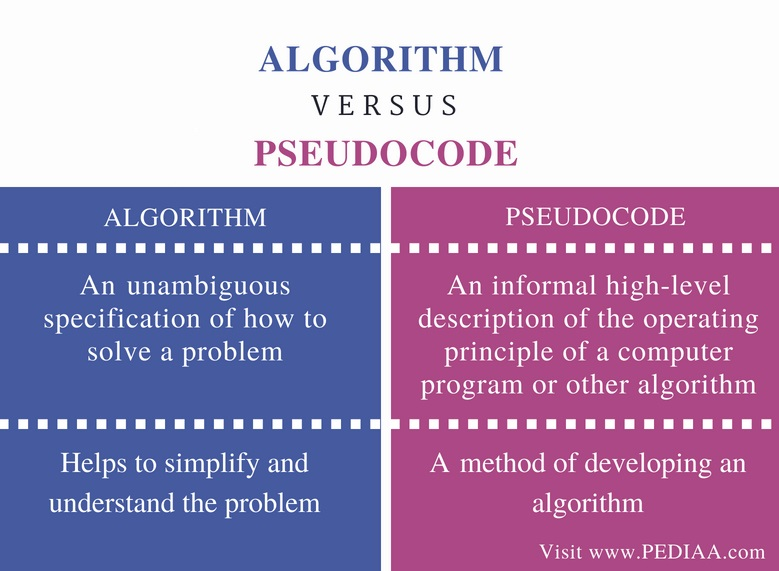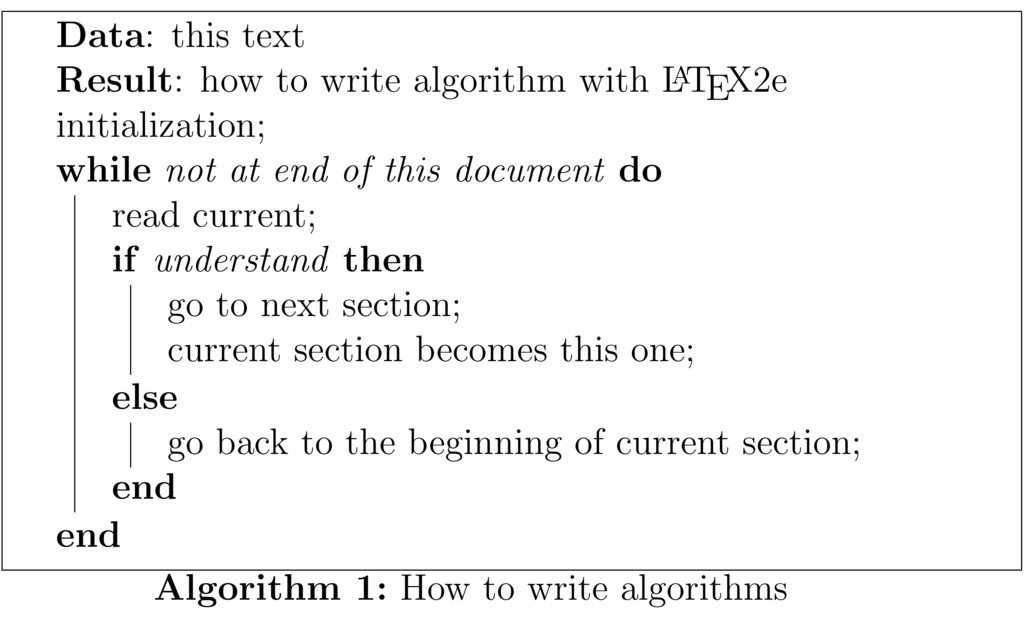# Difference Between Algorithm and Pseudocode

The main difference between algorithm and pseudocode is that an algorithm is a step by step procedure to solve a given problem while a pseudocode is a method of writing an algorithm.

An algorithm is a procedure for solving a problem. In other words, it is a sequence of steps to solve a given problem. It can contain sequences, iterations, selection, etc. Usually, there can be several methods to solve a problem. It is important to analyze each solution and select the best approach to solving it. On the other hand, a pseudocode is a method of developing an algorithm. Programmers can use informal simple language to write a pseudocode and there is no strict syntax to follow. It is a text-based detailed design tool.

### Key Areas Covered

1. What is Algorithm
– Definition, Example
2. What is Pseudocode
– Definition, Example
3. Difference Between Algorithm and Pseudocode
– Comparison of Key Differences

### Key Terms

Algorithm, Pseudocode, Programming## What is Algorithm

An algorithm is a step by step procedure to solve a problem. A procedure is a finite sequence of instructions, where each is carried out in a finite amount of time. Every problem can be solved with the help of an algorithm. For example, when the user wants to login to a Facebook account, first he has to go to Facebook.com. Then he has to give the correct username and password. Then he has to click the login button. If the username and password are correct, the user can enter his account. Likewise, every problem has a sequence of steps to solve it. This is also an algorithm because it provides a correct sequence of steps to solve the problem.Figure 1: Writing Algorithms using Pseudocode

When writing programs, it is important to identify the algorithm for the program. For example, to add two numbers, first sum variable is initialized to 0. Then two numbers are entered. Then, the addition is stored to the sum variable. Finally, the sum is printed. That is the algorithm to add two numbers.

## What is Pseudocode

Pseudocode is an informal way of writing a program. It is not exactly a computer program. It represents the algorithm of the program in natural language and mathematical notations. Usually, there is no particular code syntax to write a pseudocode. Therefore, there is no strict syntax as a usual programming language. It uses simple English language.

Pseudocode to add 2 numbers is as follows;

SumOfTwoNumbers()

Begin

Set sum=0;

Set sum = num1+num2;

Print sum;

End

Pseudocode to find the area of a Rectangle is as follows.

AreaOfRectangle()

Begin

Set area = width * length;

Print area;

End

Pseudocode of sequential flow with multiple alternatives is as follows.

DisplayValues()

Begin

if x==1 then

Print: “One”;

else if x==2 then

Print: “Two”;

else

Print: “x is not 1 or 2”;

endif

End

Pseudocode to print 5 numbers is as follows.

PrintOneToFive()

Begin

Set i=1;

while i<=5

Print : i;

Set i= i+1;

endwhile

End

Those are few examples for pseudocodes.

## Difference Between Algorithm and Pseudocode

### Definition

An algorithm is an unambiguous specification of how to solve a problem. Pseudocode is an informal high-level description of the operating principle of a computer program or other algorithm.

### Usage

An algorithm helps to simplify and understand the problem. On the other hand, pseudocode is a method of developing an algorithm.

### Conclusion

An algorithm is an arrangement of steps to solve a problem. A pseudo-code uses natural language or compact mathematical notation to write algorithms. The main difference between algorithm and pseudocode is that an algorithm is a step by step procedure to solve a given problem while a pseudocode is a method of writing an algorithm.

##### Reference:

1. Algorithm Using Flowchart and Pseudo Code Level 3 Pseudo Code, Yusuf Shakeel, 27 Aug. 2013,  Available here.

##### Image Courtesy:

1.”Latex-algorithm2e-if-else” By Lavaka – Own work (CC BY-SA 3.0) via Commons Wikimedia